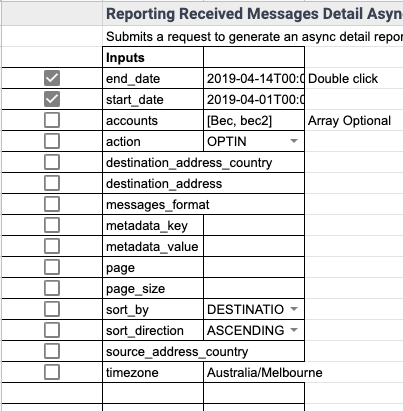# Custom reporting dashboards - Part 2

## Getting started with detail

With the detailed reporting endpoints, you can receive reporting details in your program, use advanced filtering, and then process that data any way you want to make your custom dashboard. Firstly create a filter input field. A basic one for the received messages endpoint would look like this:Down the side you can see I have made checkboxes - we’ll use those in code later to formulate the correct query string. Now in script editor, we can create our method. Go to Tools>Open Script Editor. Create a method called getReceivedMessagesDetail(). Now we can set up all the required variables for each of the checkboxes:

```var ss = SpreadsheetApp.getActiveSpreadsheet();

var end_date_full = ss.getRange("C4").getValue();
var end_date = "2019-04-30T13:30:00";
var start_date_full = ss.getRange("C5").getValue();
var start_date = "2019-04-01T13:30:00";

var accounts = ss.getRange("C6").getValue();
var action = ss.getRange("C7").getValue();
var message_format = ss.getRange("C10").getValue();
var page = ss.getRange("C13").getValue();
var page_size = ss.getRange("C14").getValue();
var sort_by = ss.getRange("C15").getValue();
var sort_direction = ss.getRange("C16").getValue();
var timezone = ss.getRange("C19").getValue();```

Now that we know whether or not a specific row is required, we can start formulating our URL, and make the API call:

```url = url + "end_date=" + end_date + "&start_date=" + start_date;

if (accounts == true){
url = url + "&accounts=" + accounts;
}
if (action == true){
url = url + "&action=" + action;
}
}
}
if (message_format == true){
url = url + "&message_format=" + message_format;
}
}
}
if (page == true){
url = url + "&page=" + page;
}
if (page_size == true){
url = url + "&page_size=" + page_size;
}
if (sort_by == true){
url = url + "&sort_by=" + sort_by;
}
if (sort_direction == true){
url = url + "&sort_direction=" + sort_direction;
}
}
}
if (timezone == true){
url = url + "&timezone=" + timezone;
}

var encoded = Utilities.base64Encode(plaintext);
var header = "Basic " + encoded;

'Content-Type': 'application/json'

};```
`var result = JSON.parse(UrlFetchApp.fetch(url, options).getContentText());`
`Logger.log(result);`

Declare your username and password variable and assign your API Key and Secret to each variable (respectively) in script editor at the top of you code, outside the method.

## Parsing and displaying the data

The data we receive back includes some pagination data and an array of all the messages that have been received during the time specified. I’m going to first display all the pagination data,by making a table like this, on our spreadsheet, and then adding some more code:```//extra code to get detail
var pagination = result.pagination;
ss.getRange("c34").setValue(pagination.page);
ss.getRange("c35").setValue(pagination.page_size);
ss.getRange("c36").setValue(pagination.total_count);
ss.getRange("c37").setValue(pagination.page_count);
ss.getRange("c38").setValue(pagination.next_uri);
ss.getRange("c39").setValue(pagination.previous_uri);```

At the bottom of our output table, we have space for the properties section of the output. The code for this is as follows:

```var properties = result.properties;
ss.getRange("c41").setValue(properties.end_date);
ss.getRange("c42").setValue(properties.start_date);
ss.getRange("c43").setValue(properties.sorting);
ss.getRange("c44").setValue(properties.filters);
ss.getRange("c46").setValue(properties.timezone);```

## Parse Message Detail

Now we can do whatever we want with the messages. The true value from this reporting endpoint will come from being able to aggregate the reporting data and produce high-level statistics, like the total number. Nonetheless, let’s look at how to cycle through all of the results and print them out on the screen for perusal:

```//message data
var data = result.data;

//define a variable for current column for printing out
var column = 5;
var rownum = 32;

//loop through each result
for each (item in data) {

// define an array of all the object keys

// define an array of all the object values
var row = headerRow.map(function(key){ return item[key]});

// define the contents of the range
var contents = [
row
];

var rng = ss.getActiveSheet().getRange(rownum, column, contents.length, headerRow.length );
rng.setValues(contents);

rownum = rownum +2;
}```

## Create data aggregates

```//create aggregated data for your dashboard
var count = Object.keys(data).length

ss.getRange("F14").setValue(count);

var responseCount = 0;
for each (item in data) {
if (item.content == "Outbreak of measles, come and get your kids"){
responseCount++;
}
}
ss.getRange("H14").setValue(responseCount);```

Since this is the responses endpoint, other data you may want to know from this reporting endpoint is, for example, the overall sentiment of the responses. You could use something like Microsoft Azure's Text Analytics API, which has a free tier, to do this kind of analysis.